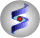ICM Manual v.3.8
by Ruben Abagyan,Eugene Raush and Max Totrov
Jun 9 2022

 Prev ICM Language Reference Transformations and symmetry Next

This section describes geometrical transformations of molecular objects and manipulations with crystallographic symmetry.

# Main concepts and functions

Molecular objects and 3D density maps may contain symmetry information. This information allows one to generate symmetry related parts of the density or molecular objects.
An elementary space transformation is defined by a rarray where values {a1,a2,...,a12} define 3x3 rotation matrix and translation vector {a4,a8,a12}. The complete augmented affine 4x4 transformation matrix in direct space can be presented as:
```
a1  a2  a3  | a4
a5  a6  a7  | a8
a9  a10 a11 | a12
------------+----
0.  0.  0.  | 1.
```
The related commands and functions (transformation vector will be referred to as R_tv):
command/functiondescription
Axis ( R_tv )calculates the rotation axis R_3 of the transformation. Rotation angle is returned in r_out .
Augment ( R_tv) converts 12-membered transformation vector into the augmented transformation matrix 4x4.
Cell ( os_ )returns {a,b,c,alpha,beta,gamma} of the unit cell
Rmsd ( as_1 as_2 [ exact ])returns R_tv in R_out
Rot ( R_tv )extracts the 3x3 rotation matrix
Rot ( R_center R_axis r_angle )returns rotation matrix for the rotate command
rotate ms_ M_rotationMatrixrotates selected molecules
superimpose as_1 as_2 ...returns R_tv in R_out
Symgroup ( i_spaceGroupNumber )returns a chain (R_[1:12*n]) of all n transformation vectors composing the specified space group.
Symgroup ( s_groupName )returns the i_spaceGroupNumber from which the transformations can be determined.
transform ms_ R_tv applies transformation to an object.
Trans ( R_tv )extracts the translation 3-vector which is applied after rotation

# How to generate symmetry related molecules

There are three steps:
• define symmetry
• find elementary transformation
• apply the transformation with the transform command.
Objects read with the read pdb or read csd commands grab the symmetry information from the files. Otherwise assign the symmetry with the set symmetry os_ s_spaceGroupName R_6cell i_NofMolecules command. This way you may define your own set of symmetry transformations. Finally, loop through all the transformations and aplly the transform command.
Example: see example in the transform command or paste the following lines into your ICM session:
```
for i=2,Nof(Symgroup(Symgroup(a_)))/12 * 2 # 2 elem.cells will be filled
copy a_1. "a"+i
transform a_a\$i. i-1
display
center
endfor
color ml a_*.
gcell=Grob("cell",Cell( ))
obl=Augment(Cell( ))          # this will work also for any oblique matrix
g1 = gcell + (-1)*obl[1:3,2]  # shift cell by a-vector
g2 = g1 + obl[1:3,3]
display gcell g1 g2
print " cell=" Cell( ) "\n Symgroup=" Symgroup(a_) \
"Nof.sym.=" Nof(Symgroup(Symgroup(a_)))/12
```

# How to find and display rotation/screw transformation axis

Steps:
• find the transformation with the Rmsd( as_sub1 as_sub2 exact) function. It returns the transformation vector in the R_out system variable.
• find the axis with the Axis( R_out) function. This function also return a point at the axis in R_out and the rotation angle in r_out
• display the axis with the Grob( "arrow", R_6 ) function.

Examples:
```
read obj "crn"  # let us display an axis of the alpha helix 7:17
ds a_/7:17
R1=Mean(Xyz(a_/7:17/ca))  # this point will be projected onto the axis
show Rmsd(a_/7:16/ca a_/8:17/ca exact ) # find the transformation
vv=R_out
aa=Axis(vv) # now R_out contains xyz of a point at the axis
print "The rotation angle is ",r_out
# grob + V3 translates grob by V3
gg=Grob("arrow",aa )*10.+ Sum((R1-R_out)*aa)*aa+R_out # projection of R1 on aa
display gg
center
pause

#Symmetry of an extended fragment
delete object
build string "se ala ala ala ala ala ala ala ala ala ala ala ala"
R1=Mean(Xyz(a_/*/ca))    # this point will be projected onto the axis
show Rmsd(a_/2:12/ca a_/1:11/ca exact )
vv=R_out
aa=Axis(vv)
print "The rotation angle is ",r_out
gg=Grob("arrow",aa )*10.+ Sum((R1-R_out)*aa)*aa+R_out # projection of R1 on aa
display gg
center
```

Now you can rotate your selection of molecules around the axis aa with the rotate command and the Rot( R_center R_axis r_angle ) function, e.g.
```
for i=0,360,30
rotate a_1 Rot( R1 aa Real(i) )
endfor
```

# How to combine several transformations

To combine several transformations simply multiply their augmented matrices.
Examples:
```
tv = Symgroup(19)   # 12*4 vector of the P212121 symmetry group
tv1 = tv[13:24]     # grab the second transformation of the symmetry group
# the first is the identity transformation
tv2 = tv[37:48]     # grab the last transformation of the symmetry group
tvComb = Vector(Augment(tv1)*Augment(tv2))      # self-explanatory
show tvComb         # you can apply it now with the transform command
```

# How to build a helix from the two contacting monomers

Two steps:
• find the transformation from the two coordinate sets
• apply the transformation as many times as you need
The transformation may be helical or (a degenerate case) a rotational.
Example:
```
display a_*.
R1=Mean(Xyz(a_/*/ca))      # this point will be projected onto the axis
show Rmsd( a_2 a_1 exact)  # we assume that molecules are the same
tv = R_out                 # this is the transformation 12-vector
ha = r_out                 # rotation angle  (just FYI)
delete a_2                 # we will regenerate the 2nd molecule anyway
rename a_1. "s1"
for i=1,4                  # build a helix with 4 new elements
j=i+1
copy a_s\$i. "s"+j        # names for a new subunits: s2,s3,s4,s5,s6
transform a_s\$j. tv      # apply tv to the last copied object
endfor
aa=Axis(tv)
print "The rotation angle is ",r_out
g_ax=Grob("arrow",aa )*10.+ Sum((R1-R_out)*aa)*aa+R_out
# projection of R1 on aa
display a_*. g_ax
color a_*. Count(Nof(a_*.))
```

 PrevImage frame by frame HomeUp NextX-ray